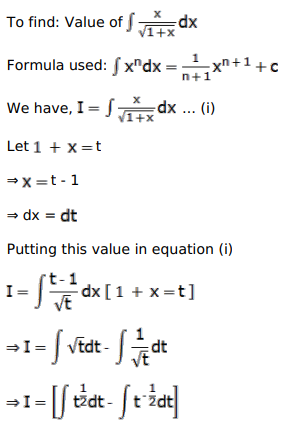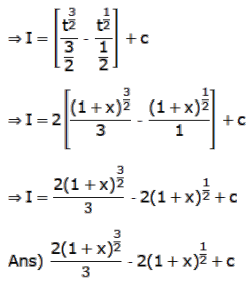# Evaluate the following integrals:

Question:

Evaluate the following integrals:

$\int \frac{x}{\sqrt{1+x}} d x$

Solution: